• 一、集合函数的概念 可以使用numpy数组实现数学上的集合运算的函数，叫做集合函数。 二、常见的集合函数 三、一些例子 引入numpy import numpy as np np.unique 返回的是数组中唯一值排序后形成的数组 import ...
一、集合函数的概念
可以使用numpy数组实现数学上的集合运算的函数，叫做集合函数。
二、常见的集合函数三、一些例子
引入numpy
import numpy as np


np.unique  返回的是数组中唯一值排序后形成的数组

import numpy as np
# np.unique，返回的是数组中唯一值排序后形成的数组
names =np.array(['Bob', 'Joe', 'Will', 'Bob', 'Will', 'Joe', 'Joe'])
print("****************")
print(np.unique(names))

结果
****************
['Bob' 'Joe' 'Will']


np.in1d  可以检查一个数组中的值是否在另外一个数组中，并返回一个布尔值数组：

values = np.array([6, 0, 0, 3, 2, 5, 6])
print("*****************")
print(np.in1d(values, [2, 3, 6]))

结果
*****************
[ True False False  True  True False  True]



展开全文python numpy
• 这次要修炼目标是[集合函数概念]。 正剧开始： 星历2016年04月08日 11:41:49, 银河系厄尔斯星球中华帝国江南行省。 [工程师阿伟]正在和[机器小伟]一起研究[集合函数概念]。 ...
剧情提要：[机器小伟]在[工程师阿伟]的陪同下进入了结丹初期的修炼，这次要修炼的目标是[集合与函数概念]。
正剧开始：
星历2016年04月08日 11:41:49, 银河系厄尔斯星球中华帝国江南行省。
[工程师阿伟]正在和[机器小伟]一起研究[集合与函数概念]。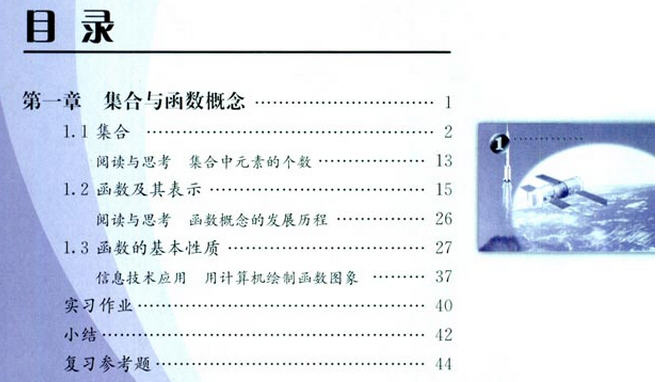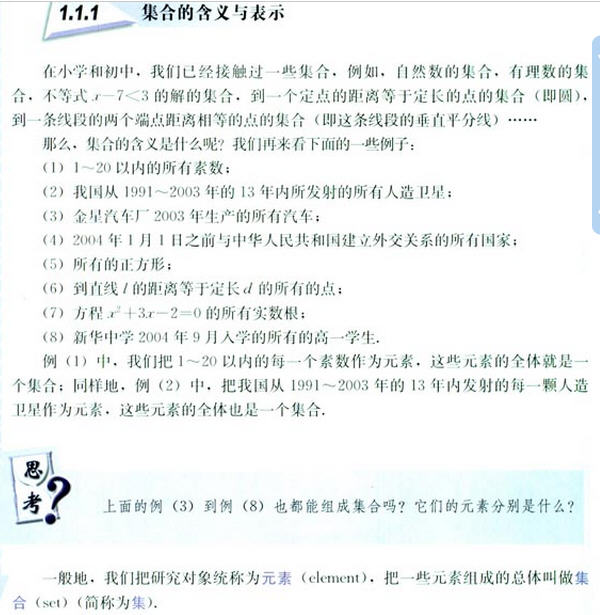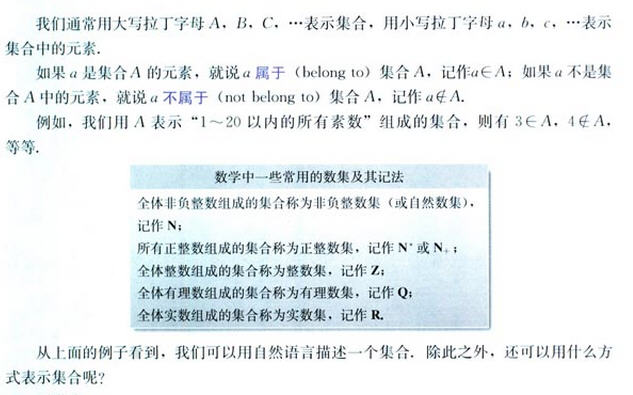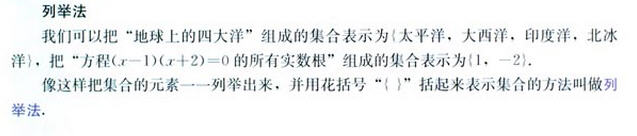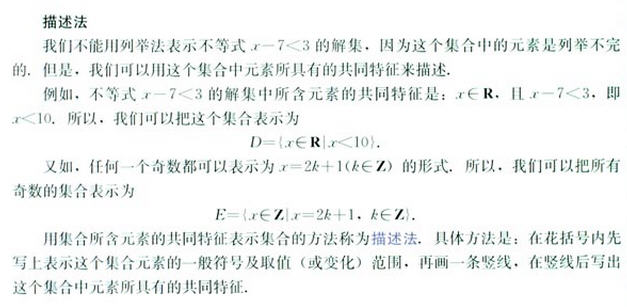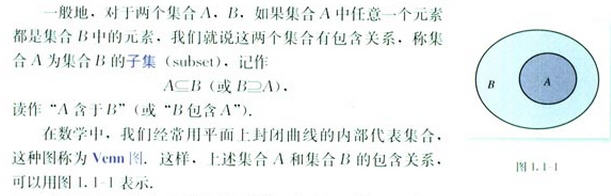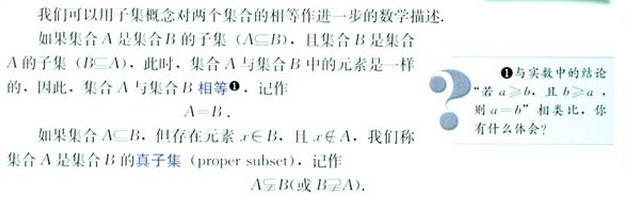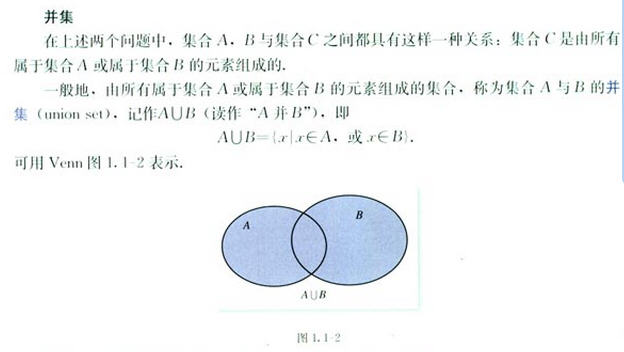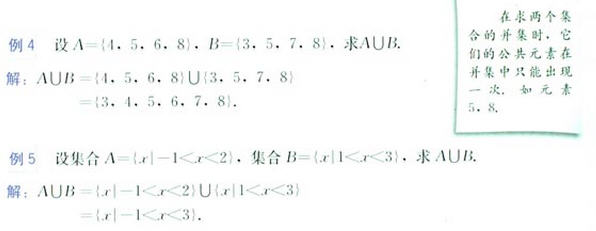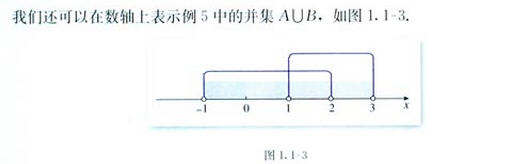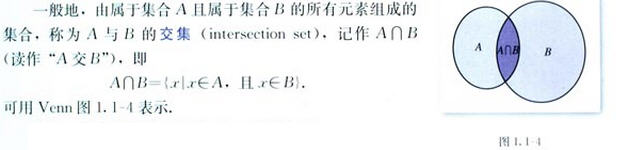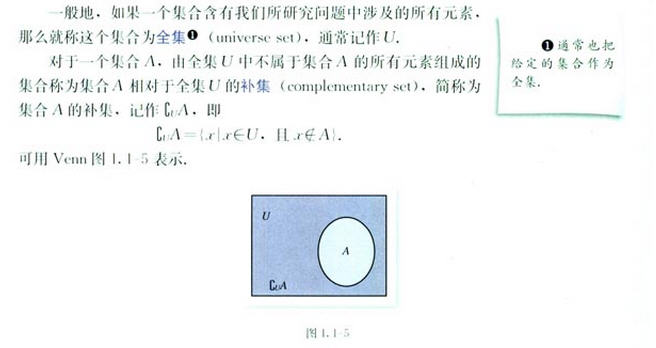小伟看到这里的时候，是一阵的头晕目眩哪，这些稀奇古怪的符号，是从哪儿冒出来的啊
。而且，如果不能很容易的写出它们，看来是没法进行下去了。

为了找出怎样写出这些符号，小伟找啊找，终于找到了。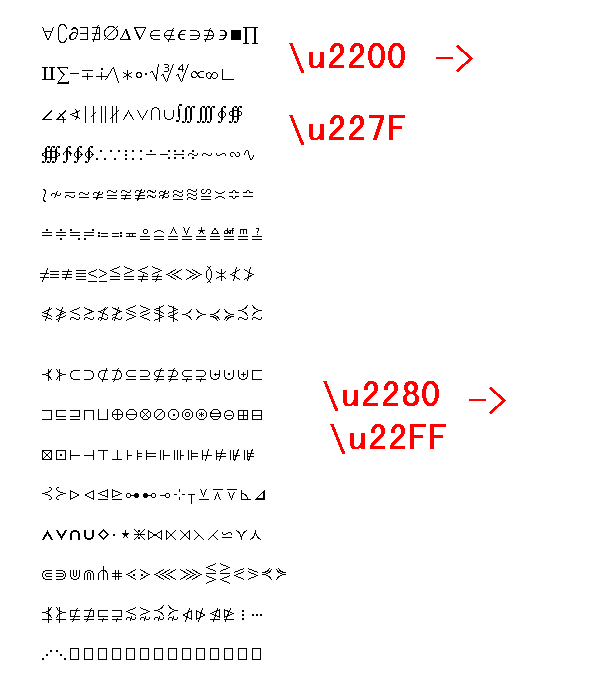<span style="font-size:18px;">	if (1) {
var r = 20;
/*
config.setSector(1,1,1,1);
config.graphPaper2D(0, 0, r);
config.axis2D(0, 0, 190);*/

var a = '0123456789ABCDEF';

var x = y = 0;
var s = '';

for (var i = 0; i < 16; i++) {
s = '';

for (var j = 0; j < 16; j++) {

s += '\\'+'u22'+a[i]+a[j];

}
document.write('\''+s+'\','+'<br/>');

}

}

if (1) {

var s = [
'\u2200\u2201\u2202\u2203\u2204\u2205\u2206\u2207\u2208\u2209\u220A\u220B\u220C\u220D\u220E\u220F',
'\u2210\u2211\u2212\u2213\u2214\u2215\u2216\u2217\u2218\u2219\u221A\u221B\u221C\u221D\u221E\u221F',
'\u2220\u2221\u2222\u2223\u2224\u2225\u2226\u2227\u2228\u2229\u222A\u222B\u222C\u222D\u222E\u222F',
'\u2230\u2231\u2232\u2233\u2234\u2235\u2236\u2237\u2238\u2239\u223A\u223B\u223C\u223D\u223E\u223F',
'\u2240\u2241\u2242\u2243\u2244\u2245\u2246\u2247\u2248\u2249\u224A\u224B\u224C\u224D\u224E\u224F',
'\u2250\u2251\u2252\u2253\u2254\u2255\u2256\u2257\u2258\u2259\u225A\u225B\u225C\u225D\u225E\u225F',
'\u2260\u2261\u2262\u2263\u2264\u2265\u2266\u2267\u2268\u2269\u226A\u226B\u226C\u226D\u226E\u226F',
'\u2270\u2271\u2272\u2273\u2274\u2275\u2276\u2277\u2278\u2279\u227A\u227B\u227C\u227D\u227E\u227F',
'\u2280\u2281\u2282\u2283\u2284\u2285\u2286\u2287\u2288\u2289\u228A\u228B\u228C\u228D\u228E\u228F',
'\u2290\u2291\u2292\u2293\u2294\u2295\u2296\u2297\u2298\u2299\u229A\u229B\u229C\u229D\u229E\u229F',
'\u22A0\u22A1\u22A2\u22A3\u22A4\u22A5\u22A6\u22A7\u22A8\u22A9\u22AA\u22AB\u22AC\u22AD\u22AE\u22AF',
'\u22B0\u22B1\u22B2\u22B3\u22B4\u22B5\u22B6\u22B7\u22B8\u22B9\u22BA\u22BB\u22BC\u22BD\u22BE\u22BF',
'\u22C0\u22C1\u22C2\u22C3\u22C4\u22C5\u22C6\u22C7\u22C8\u22C9\u22CA\u22CB\u22CC\u22CD\u22CE\u22CF',
'\u22D0\u22D1\u22D2\u22D3\u22D4\u22D5\u22D6\u22D7\u22D8\u22D9\u22DA\u22DB\u22DC\u22DD\u22DE\u22DF',
'\u22E0\u22E1\u22E2\u22E3\u22E4\u22E5\u22E6\u22E7\u22E8\u22E9\u22EA\u22EB\u22EC\u22ED\u22EE\u22EF',
'\u22F0\u22F1\u22F2\u22F3\u22F4\u22F5\u22F6\u22F7\u22F8\u22F9\u22FA\u22FB\u22FC\u22FD\u22FE\u22FF'
];

var x =40, y=40;
var r1 = 40;

var len = s.length;
for (var i = 0; i < 8; i++) {
plot.fillText(s[i], x, y, 500);
y+=r1;

}
}
</span>
于是，阿伟帮助小伟又进化了一下以前写表达式的工具，这下可以写。先看看效果吧。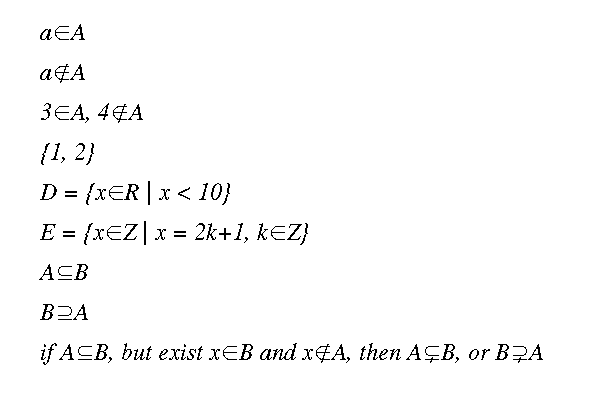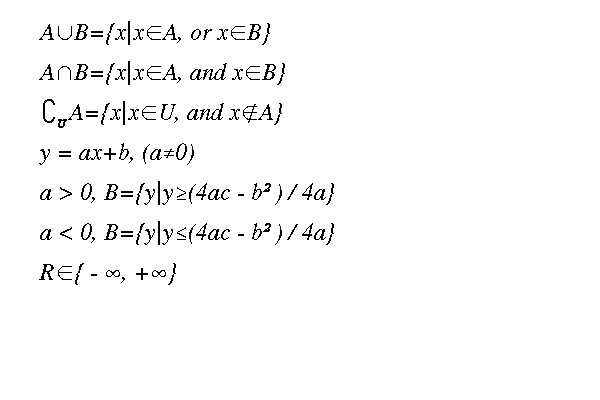这是怎么写出来的啊，困不困难呀。
小伟是这样写的：

<span style="font-size:18px;">	var s = [
/*
'a[B]A',
'a[NB]A',
'3[B]A, 4[NB]A',
'{1, 2}',
'D = {x[B]R | x < 10}',
'E = {x[B]Z | x = 2k+1, k[B]Z}',
'A[S]B',
'B[SS]A',
'if A[S]B, but exist x[B]B and x[NB]A, then A[ST]B, or B[SST]A',*/

'A[UU]B={x|x[B]A, or x[B]B}',
'A[I]B={x|x[B]A, and x[B]B}',
'[C]_[U]A={x|x[B]U, and x[NB]A}',
'y = ax+b, (a!=0)',
'a > 0, B={y|y>=(4ac-b^)/4a}',
'a < 0, B={y|y<=(4ac-b^)/4a}',
'R[B]{-[INF], +[INF]}',

];</span>
虽然看起来像是天书差不多，不过好在可以用简便一点的方法来写那些稀奇符号了。
这里是对照表：

<span style="font-size:18px;">		if (s != '') {
s = s.replace(/$B$/ig, '\u2208'); //∈
s = s.replace(/$NB$/ig, '\u2209'); //不属于
s = s.replace(/$S$/ig, '\u2286'); //包含于(是子集)
s = s.replace(/$SS$/ig, '\u2287'); //包含
s = s.replace(/$ST$/ig, '\u228A'); //真包含于(是真子集)
s = s.replace(/$SST$/ig, '\u228B'); //真包含

s = s.replace(/$UU$/ig, '\u222A'); //并集 ，由于U表示全集，又常为下标，此处错开
s = s.replace(/$I$/ig, '\u2229'); //交集
s = s.replace(/$C$/ig, '\u2201'); //补集
s = s.replace(/$INF$/ig, '\u221E'); //无穷大

}</span>
然后这里是工具：
<span style="font-size:18px;">/**
* @usage   数学表达式，代数式的书写
* @author  mw
* @date    2016年03月12日  星期六  11:05:12
* @param
* @return
*
*/
function MathText() {
//上标标记形式为...^[内容]...
//分数不进行处理， 根式不进行处理，都转成指数式进行
//特殊数学符号设想加$alpha]进行转义，待续 //可以进行指数上标代数式的书写 //可扩展下标，待续 this.setNormalFont = function() { plot.setFont("normal normal normal 24px Times Lt Std"); } this.setScriptFont = function() { plot.setFont("italic normal bold 16px Dark Courier "); } this.print = function(text, xPos, yPos) { xPos = xPos ? xPos : 0; yPos = yPos ? yPos : 0; plot.save(); var s = text ? text : ''; if (s != '') { s = s.replace(/\/\//ig, '÷'); s = s.replace(/>=/ig, '≥'); s = s.replace(/<=/ig, '≤'); s = s.replace(/!=/ig, '≠'); s = s.replace(/pi/ig, 'π'); } //字符串长度 var len = s.length; //不同字体大小设置在此 var r1 = 20; //单个字符暂存处 var c; //文本显示位置 var x = xPos, y = yPos; //正常文本暂存 var s0 = ''; //字符串打印长度 var measure; //记录上一个x位置,可记录三层 var xMem = [x, x, x]; //记录每一层的左括号位置 var bracketPos = [x, x, x]; //记录括号层次 var bracketsLevel = 0; //记录根号层次 var radicalLevel = 0; //记录每一层根号的起始位置和层次数的数组...[[start, end, level], ...] var radicalSpan = []; //设置正常字体 this.setNormalFont(); for (var i = 0; i < len; i++) { if (s[i] == '_') { //下标开始 //下标标记形式为..._[内容]... if (s0 != '') { //先把正常字符打印出 if (r1 != 20) { //字体字号大小还在上标状态 r1 = 20; this.setNormalFont(); } measure = plot.measureText(s0); plot.fillText(s0, x, y, measure); s0 = ''; x += measure; } var subScript = ''; var j = 0; for (j = i+1; s[j]!=']'; j++) { if (s[j] != '[') { subScript+=s[j]; } } if (r1 != 10) {//正常字体状态，需要改为上标字体 r1 = 10; this.setScriptFont(); } measure = plot.measureText(subScript); plot.fillText(subScript, x, y+8, measure); if (j < len-1 && s[j+1] == '^') { } else { x += 1.2*measure; } i = j; } else if (s[i] == '^') { //上标开始 //上标标记形式为...^[内容]... if (s0 != '') { //先把正常字符打印出 if (r1 != 20) { //字体字号大小还在上标状态 r1 = 20; this.setNormalFont(); } measure = plot.measureText(s0); plot.fillText(s0, x, y, measure); s0 = ''; x += measure; } var upperScript = ''; var j = 0; for (j = i+1; s[j]!=']'; j++) { if (s[j] != '[') { upperScript+=s[j]; } } //二次根式 if (upperScript == '1/2' || upperScript == '0.5') { var x1, y1; if (i > 0 && s[i-1] == ')') { x1 = bracketPos[bracketsLevel]; } else { x1 = xMem[bracketsLevel]; } /* 存疑代码 if (radicalSpan == []) { radicalLevel = 0; radicalSpan.push([x1, x, radicalLevel]); } else { var len = radicalSpan.length; for (var k = 0; k < len; k++) { if (x1 < radicalSpan[k]) { radicalLevel = radicalSpan[k]+1; break; } if (k >= len-1) { radicalLevel = 0; } } radicalSpan.push([x1, x, radicalLevel]); }*/ y1 = y-20-5*radicalLevel; plot.save() .setLineWidth(1); plot.beginPath() .moveTo(x1-5, y+5) .lineTo(x1-8, y-3) .moveTo(x1-5, y+5) .lineTo(x1+5, y1) .moveTo(x1+5, y1) .lineTo(x, y1) .closePath() .stroke(); plot.restore(); } else { if (r1 != 10) {//正常字体状态，需要改为上标字体 r1 = 10; this.setScriptFont(); } measure = plot.measureText(upperScript); plot.fillText(upperScript, x, y-8, measure); if (j < len-1 && s[j+1] == '_') { } else { x += 1.2*measure; } } //直接跳跃过上标字符区段 i = j; } else { c = s[i]; if (c == ')') { s0 += c; bracketsLevel -= 1; } else if (c == '(') { //如果整个括号被开根式，根号在括号左边 bracketPos[bracketsLevel] = x + plot.measureText(s0); s0 += c; bracketsLevel+=1; //过了括号就是过了一道关，要刷新坐标 xMem[bracketsLevel] = x + plot.measureText(s0); } else if (c == '+' || c == '-' || c == '*' || c == '/' || c == '÷' || c == '=' || c == ' ') { if (c == '*') { if (i > 0 && /[0-9]/.test(s[i-1]) && /[0-9]/.test(s[i+1])) { //对于乘号前后都是数字的情况，把乘号改成叉号 c = ' \u00D7 '; } else { //对于代数式中，乘号改为点号 c = ' \u00B7 '; } } //如果是运算符后的数被开根式，根号在运算符右边 if (c == '-' || c == '/') { s0 += ' '+c+' '; } else { s0 += c; } if (bracketsLevel < 3) { xMem[bracketsLevel] = x+plot.measureText(s0); } } else { s0 += c; } } } if (s0 != '') { //先把正常字符打印出 if (r1 != 20) { //字体字号大小还在上标状态 r1 = 20; this.setNormalFont(); } measure = plot.measureText(s0); plot.fillText(s0, x, y, measure); x += measure; } plot.restore(); } //集合符号，集合表达式的书写 this.printSet = function(text, xpos, ypos) { var s = text ? text : ''; if (s != '') { s = s.replace(/\[B$/ig, '\u2208'); //∈
s = s.replace(/$NB$/ig, '\u2209'); //不属于
s = s.replace(/$S$/ig, '\u2286'); //包含于(是子集)
s = s.replace(/$SS$/ig, '\u2287'); //包含
s = s.replace(/$ST$/ig, '\u228A'); //真包含于(是真子集)
s = s.replace(/$SST$/ig, '\u228B'); //真包含

s = s.replace(/$UU$/ig, '\u222A'); //并集 ，由于U表示全集，又常为下标，此处错开
s = s.replace(/$I$/ig, '\u2229'); //交集
s = s.replace(/$C$/ig, '\u2201'); //补集
s = s.replace(/$INF$/ig, '\u221E'); //无穷大

}

return this.print(s, xpos, ypos);
}

}</span>

这里是调用：

<span style="font-size:18px;">	if (1) {
var mathText = new MathText();

var s = [
/*
'a[B]A',
'a[NB]A',
'3[B]A, 4[NB]A',
'{1, 2}',
'D = {x[B]R | x < 10}',
'E = {x[B]Z | x = 2k+1, k[B]Z}',
'A[S]B',
'B[SS]A',
'if A[S]B, but exist x[B]B and x[NB]A, then A[ST]B, or B[SST]A',*/

'A[UU]B={x|x[B]A, or x[B]B}',
'A[I]B={x|x[B]A, and x[B]B}',
'[C]_[U]A={x|x[B]U, and x[NB]A}',
'y = ax+b, (a!=0)',
'a > 0, B={y|y>=(4ac-b^)/4a}',
'a < 0, B={y|y<=(4ac-b^)/4a}',
'R[B]{-[INF], +[INF]}',

];

var x =40, y=40;
var r1 = 40;

var len = s.length;
for (var i = 0; i < len; i++) {

if (s[i] == '') {
if (x < 100) {
x += 300;
y-=r1*3;
}
else {
x = 20;
y += r1;
}
}
else {
mathText.printSet(s[i], x, y);
y+=r1;
}
}

}</span>
好了，至少现在书写难题解决了。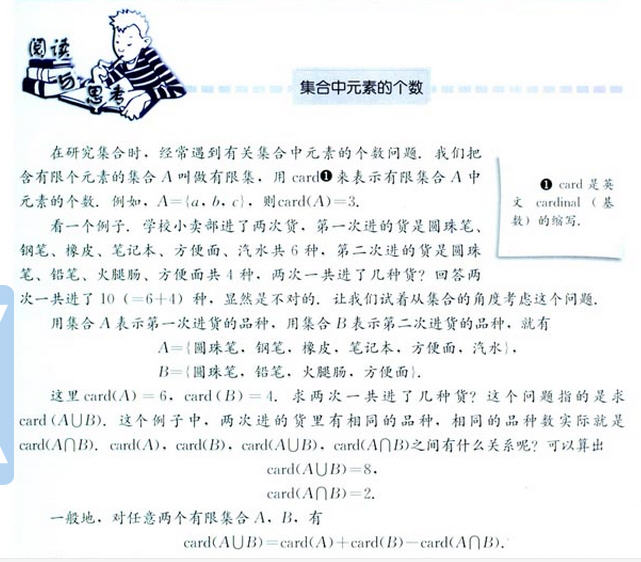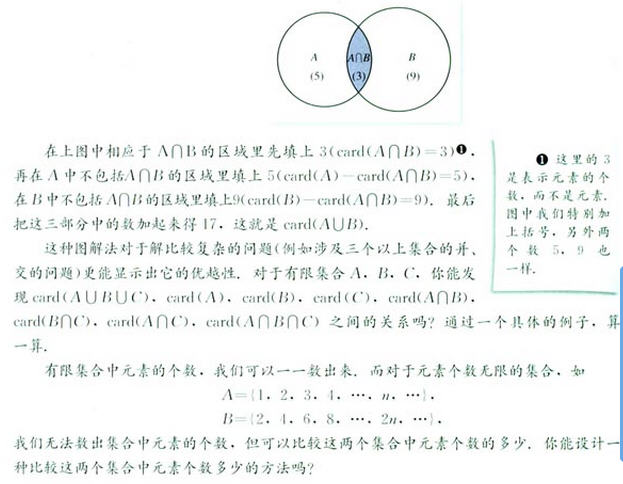这个问题好办，取10000个数作为范围，可以知道A有10000， B有5000。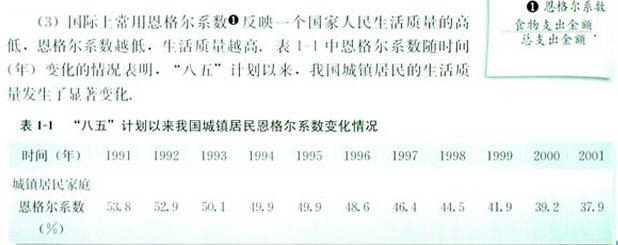这个恩氏系数小伟还是蛮关心的，先看一下这些数据的直观图吧：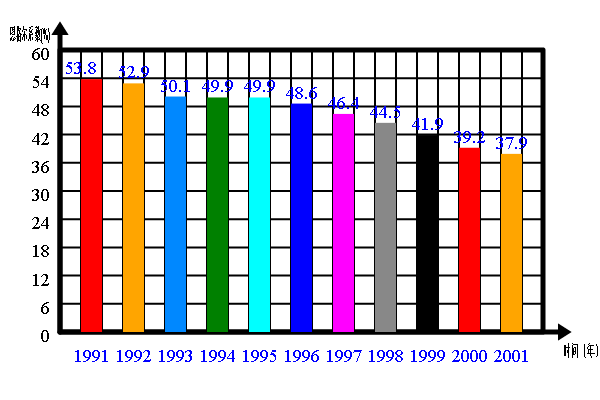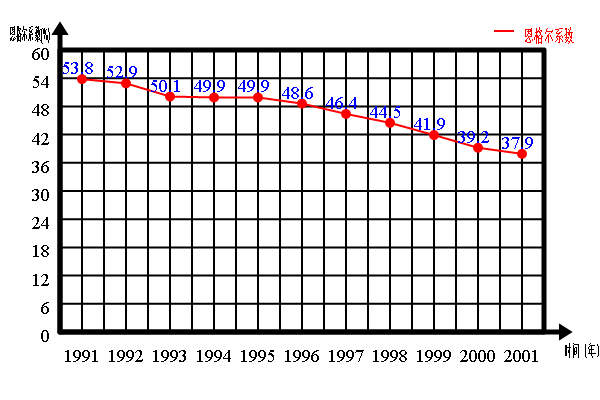这些数据呢，小伟看不懂，不过感觉很牛的样纸。
<span style="font-size:18px;">	if (1) {
var stat = new Statistic();
var data = [53.8,52.9,50.1,49.9,49.9,48.6,46.4,44.5,41.9,39.2,37.9];
var text = [];

for (var i = 1991; i <= 2001; i++) {
text.push(i.toFixed(0));
}

stat.init(data, '时间（年）', '恩格尔系数(%)', 1);
stat.barChart(text, 0, 0);

}

if (1) {
var stat = new Statistic();
var data = [53.8,52.9,50.1,49.9,49.9,48.6,46.4,44.5,41.9,39.2,37.9];
var text = [];
var size = data.length;

//为了绘制折线图，要把一维数组变化成二维数组
for (var i = 0; i < size; i++) {
data.push([data.shift()]);
}

for (var i = 1991; i <= 2001; i++) {
text.push(i.toFixed(0));
}

stat.init(data, '时间（年）', '恩格尔系数(%)', 1);
stat.multiLineGraph(text, ['恩格尔系数'], 0, 0);

}</span>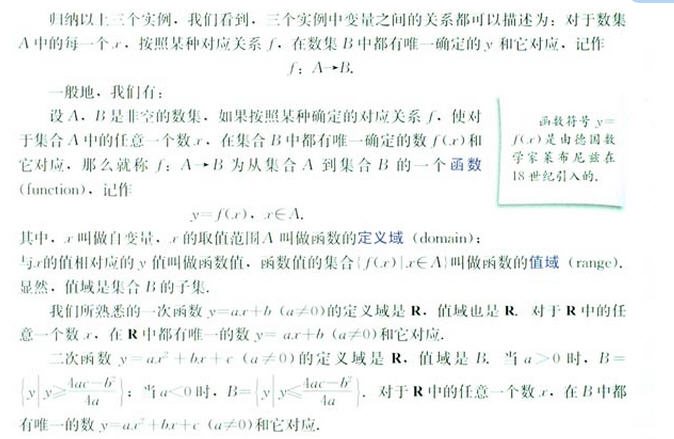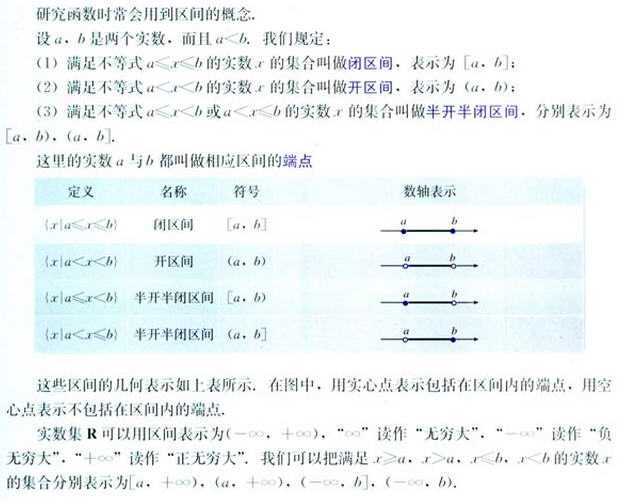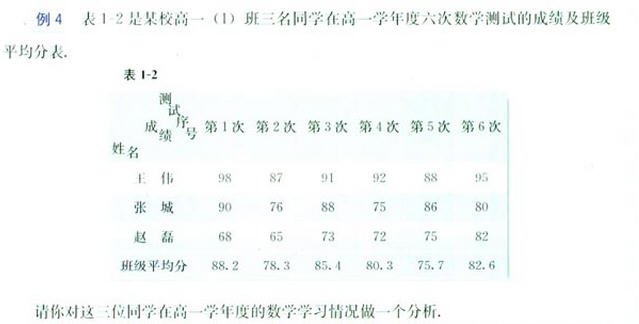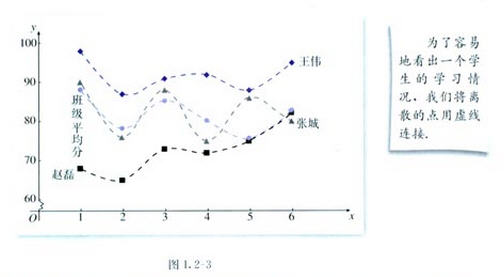这几位同学的成绩到底怎样啊，小伟决定看一看。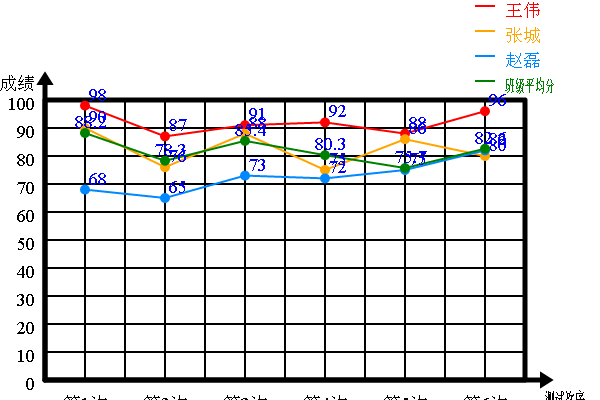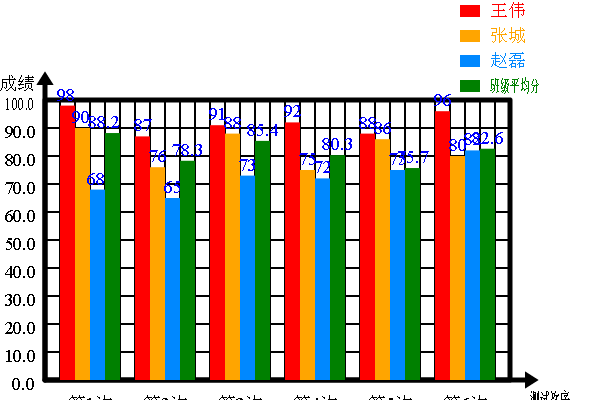看来这个和小伟同名的同学也是一位学霸啊，每次都考这么多分。

<span style="font-size:18px;">		if (1) {
var stat = new Statistic();
var wangWei = [98,87,91,92,88,96],
zhangChen = [90,76,88,75,86,80],
zhaoLei = [68,65,73,72,75,82],
average = [88.2,78.3,85.4,80.3,75.7,82.6];
var text = [];
var size = average.length;
var data = [];

//为了绘制折线图，要把一维数组变化成二维数组
for (var i = 0; i < size; i++) {
data.push([wangWei[i], zhangChen[i], zhaoLei[i], average[i]]);
}

for (var i = 1; i <= 6; i++) {
text.push('第'+i.toFixed(0)+'次');
}

stat.init(data, '测试次序', '成绩', 4);
stat.multiBarChart(text, ['王伟', '张城', '赵磊', '班级平均分'], -15, 50);
//stat.multiLineGraph(text, ['王伟', '张城', '赵磊', '班级平均分'], -15, 50);

}
</span>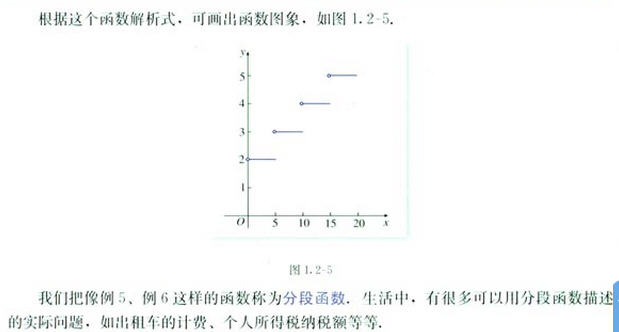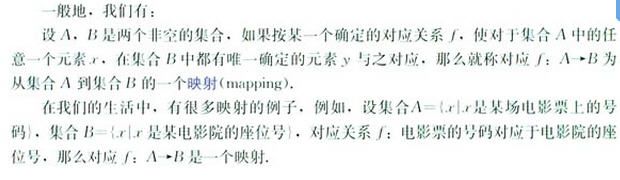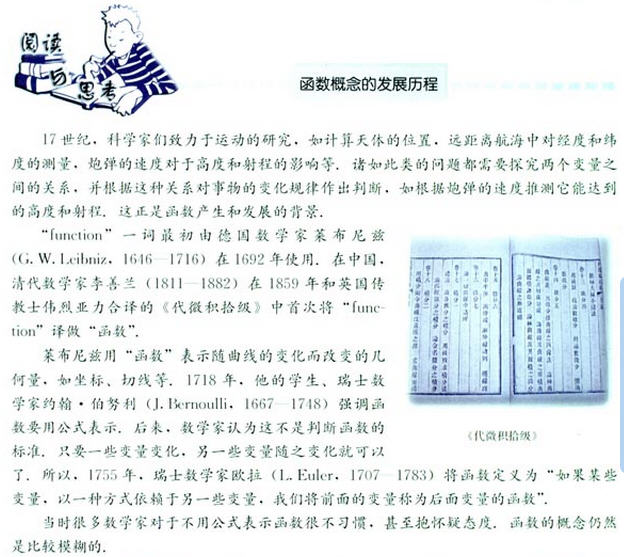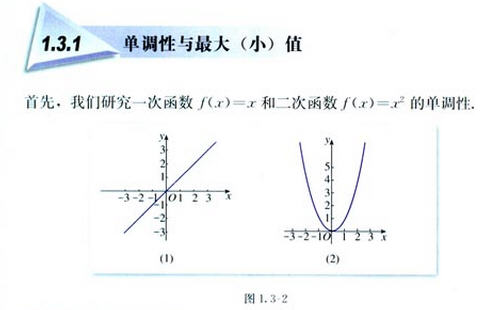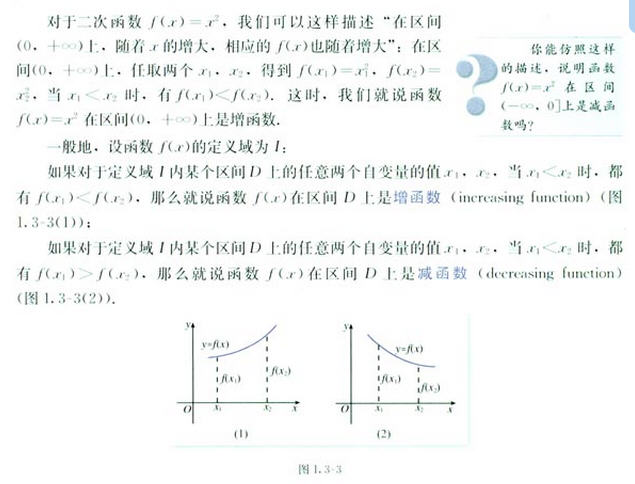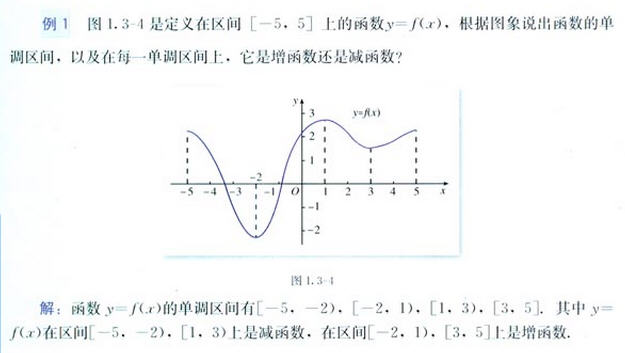小伟做了一个求单调性的小工具：
<span style="font-size:18px;">//判断单调性
//单调性
//[词典]	monotonicity; monotone; monotonic; monotony;
function monotonicityJudge(array, range) {
//传入参数
//array 是[[x1, y1], [x2, y2], ...]的映射对格式
//range 是需要判断的区间[minX, maxX]

var size = array.length;

if (size < 1 || array.length < 2)
return false;

array.sort(function(a, b) {
return (a-b);
});

var minX = range < range ? range : range,
maxX = range < range ? range : range;

var y1 = y2 = y3 = 0;

for (var i = 1; i < size-1; i++) {
if (array[i] >= minX && array[i] <= maxX) {
y1 = array[i-1];
y2 = array[i];
y3 = array[i+1];

//在给定区间中不存在单调性
if ((y1-y2)*(y2-y3)< 0)
return [false, ''];
}

if (array[i] > maxX)
break;
}

if (y3 < y2) {
return [true, '单调递减'];
}
else {
return [true, '单调递增'];
}
}</span>
不过好像不是很好玩。

<span style="font-size:18px;">	if (1) {
var array = [];
var x = y = 0;

for (var i = -10; i<=10; i+=1) {
x = i;
y = 1/x;

if (y) {
array.push([x, y]);
}
}

var range = [-3, -2];
var minX = range < range ? range : range,
maxX = range < range ? range : range;

var result = monotonicityJudge(array, range);
if (result == true) {
document.write('数组（映射）在区间'+minX.toFixed(2)+'到'
+maxX.toFixed(2)+'上具有单调性，'+result+'<br/>');

}
else {
document.write('数组（映射）在区间'+minX.toFixed(2)+'到'
+maxX.toFixed(2)+'上不具有单调性。'+'<br/>');
}

}</span>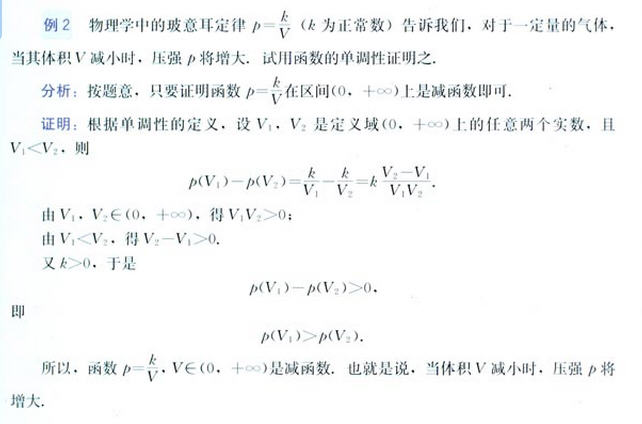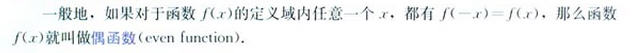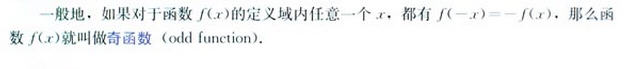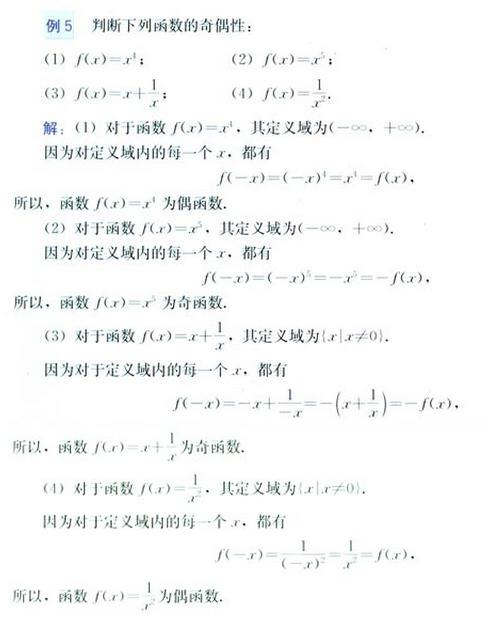这个是求奇偶性的小工具。
<span style="font-size:18px;">if (1) {
var array = [];
var x = y1 = y2 = 0;
var result = [];

var range = 10000;
var begin = 0;

for (var i = 0; i < 10; i++) {
x = begin+Math.round(Math.random()*range)%range;

//函数写在这里
y1 = 1/(x*x);
x = -x;
y2 = 1/(x*x);

if (y1 == y2) {
result.push(1);
}
else if (y1 == -y2) {
result.push(-1);
}
else {
result.push(0);
}
}

var count = 0;
var len = result.length;

for (var i = 0; i < len; i++) {
count += result[i];
}

if (count == len) {
document.write('是偶函数'+'<br/>');
}
else if (count == -len) {
document.write('是奇函数'+'<br/>');
}
else {
document.write('是非奇非偶函数'+'<br/>');
}

}

}</span>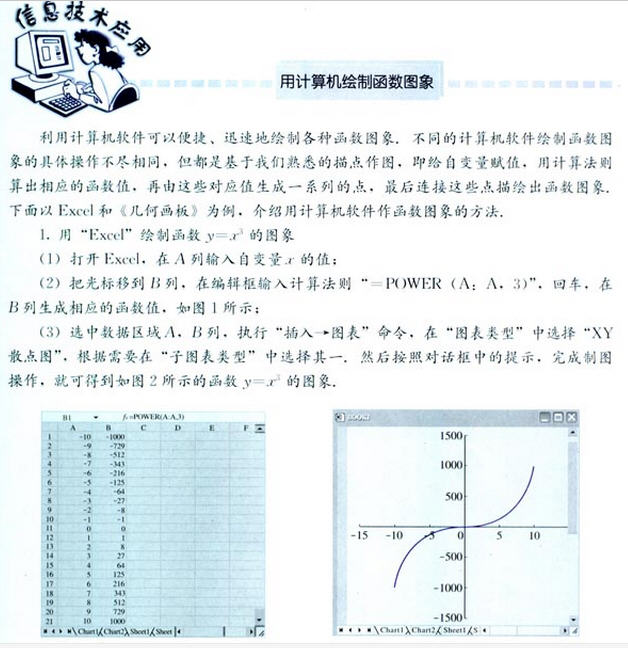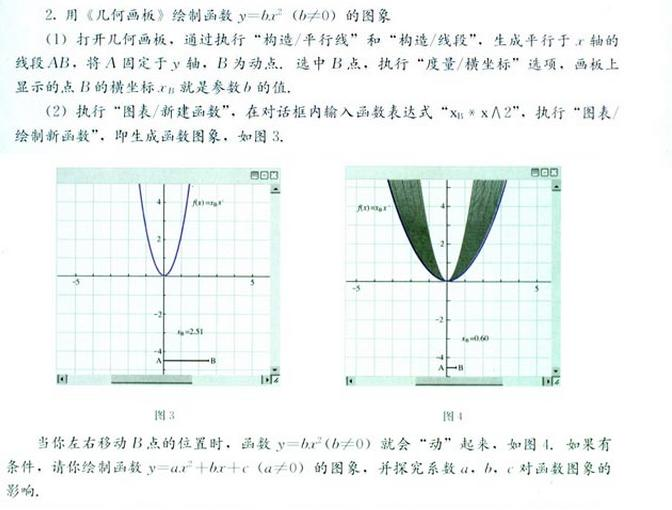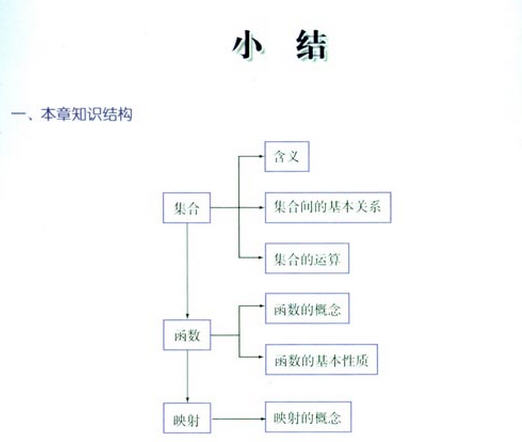本节到此结束，欲知后事如何，请看下回分解。

展开全文• 一、函数的概念 1、函数概念：代码集合，完成某种特定功能。一次编写，多次调用 2、函数定义： function 函数名（参数1，参数2…）{ 语句 }
一、函数的概念
1、函数概念：代码集合，完成某种特定功能。一次编写，多次调用
2、函数定义：
function 函数名（参数1，参数2…）{
语句
}


展开全文• 1.函数就是完成特定功能代码的集合，这组代码可以作为一个单位使用，并且给他取一个名字。 可以通过函数名在程序不同地方多次执行。（函数调用） 2.为什么要使用函数？ （1）降低编程难度。 通常将一个复杂...
一、什么叫函数
1.函数就是完成特定功能的代码的集合，这组代码可以作为一个单位使用，并且给他取一个名字。
可以通过函数名在程序的不同地方多次执行。（函数调用）
2.为什么要使用函数？
（1）降低编程的难度。
通常将一个复杂的大过程分解成n个小过程，然后将小过程划分成更小的过程，当过程细化为足够简单时，我们就可以分而治之。各个小过程解决了，大过程就迎刃而解了。
（2）代码复用
避免重复编写某段代码，提高效率。
二、定义函数
1.函数的定义和调用
def 函数名([参数列表（形参）]):                  #定义函数
#函数体
#return返回值
函数名([参数列表（实参）])                       #调用函数

函数名的命令也有规则，和变量一样
1.只能是一个词。
2.只能包含字母、数字、和下划线。
3.不能以数字开头。
4.不可以使用关键字。
5.取名尽量有意义
案例如下：
计算n以内的奇数的积：
def ji(n):
index = 0
sum1 = 1
while index <= n:
if index % 2 == 1:
sum1 *= index
index += 1
return sum1
n= int(input("请输入一个数："))
a=ji(n)
print("%s以内的奇数的积：%s" %(n,a))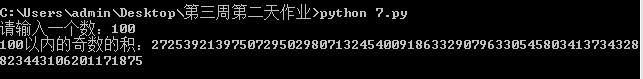三、函数的分类
任何事的分类都是根据某一标准进行分类的，下面我们根据几个条件将函数分为几类

通过有无参数
1.有参函数
2.无参函数

通过有无返回值
1.有返回值的函数
2.无返回值的函数

通过定义者
1.系统函数
2.自定义函数
3.第三方定义的函数


展开全文• ## 函数的概念及功能

千次阅读 2018-09-25 20:30:09
函数名（是调用这个函数的一个依据） 参数（是函数实现功能时要用到的必要数据）  返回值（函数运算结果）  功能（函数的功能是什么，下面会给出具体的阐述）   函数是一个自我包含的完成一定相关功能的...
• Python – 函数专题–函数的基本概念 函数就是将一些语句集合在一起的模块，他们能够不止一次的在程序中运行。函数还可以计算出一个返回值，并能够改变函数输入的参数，而且这些参数在代码的运行中每次都不会相同。 ...
• 复习引入： ...将自变量x取值的集合叫做函数的定义域，和自变量x的值对应的y值叫做函数值， 函数值的集合叫做函数的值域. 初中已经学过： 正比例函数 y=kx （k≠0） 一次函数 y=kx+b （k...
• 模块是处理某一类问题的函数和类的集合。 2 包就是一个完成特定任务工具箱。 3 包必须含有一个__init__.py文件，它用于标识当前文件夹是一个包。 4 python程序是由一个个模块组成。模块把一组相关的函数或...
• 文章目录集合集合的概念集合的基本操作添加元素移除元素计算集合元素个数清空集合判断元素是否在集合中存在交集 查看重复的元素并集差集异或集函数定义一个函数语法计算面积函数:函数调用参数传递可更改(mutable)与...
• 文章目录前言一、函数的定义二、函数的返回值三 函数的传参 前言 Linux Shell编程中也会使用到函数，函数可以把大的命令集合分解成若干较小的任务，也避免重复编写相同的代码。在Shell中，所有的函数定义都是平行的...shell
• 2019年高中数学 1.2.1.1函数的概念导学案 新人教A版必修1 一、教学目标：1. 通过丰富实例，进一步体会函数是描述变量之间的依赖关系的重要数学模型，在此基础上学习用集合与对应的语言来刻画函数
• 1、数组的概念 数字（Array），可以把一组相关的数据一起存放，并提供方便的访问（获取）方式 数组是指：一组数据的集合，其中的每个数据被称作元素，在数组中可以存放任意类型的元素 数组是一种将一组数据存储在...
• 函数 1.什么是函数 函数就是功能、过程。具有特定功能的代码的集合，这段代码是...函数的名称定义和变量的命名规则保持一致！ def 函数名称([参数列表...]): #这里的参数为形参 # 函数体 # return 返回值 3.函数...python lambda
• Z中模糊集合A由隶属度函数pa（z）老描述，它是与Z中每个元素相关联位于区间【0,1】内实数。对于来自Z某个具体元素Z0，pa（z0）值表示A中z0隶属度等级。 对于普通集合，我们说某个元素属于或者不属于集合...
• 一、关于函数的概念函数的概念有两个，其一为初中的定义，称为传统定义，其二为高中的定义，称为近代定义。 传统定义：设在某变化过程中有两个变量$$x$$、$$y$$，如果对于$$x$$在某一范围内的每一个确定的值，\(y...
• 函数的定义 《集合论》 常见数集的集合 集，合的表示方法：列举法和描述法 用描述法表示时候要有相当明确（清晰）的概念。 空集是任何元素的子集， 记 ∅∅\varnothing 补集，都带“相当于XX集”来说，集合A...
• 3.函数的组成 function：用于定义一个函数； 函数名：一个合法的标识符，不能是保留字或表达式 参数:可以有0个、1个或多个参数； 形参parameter：函数定义的时候在小括号里面定义的参数列表。用来接收实际参数。 ...js javascript
• 【集合】集合起来的一组数据。和它类似的概念：数组 【集合与数组的区别】1.数组是类型相同的一组数据，而集合中的数据类型可以相同，也...【集合函数-List】都是接口(即抽象函数的最高实现）,目的就是为了被实现...
• Python – 函数专题–函数的基本概念 函数就是将一些语句集合在一起的模块，他们能够不止一次的在程序中运行。函数还可以计算出一个返回值，并能够改变函数输入的参数，而且这些参数在代码的运行中每次都不会相同。 ...python
• 函数式接口 概念 函数式接口在Java中是指：有且仅有一个抽象方法接口。 函数式接口，即适用于函数式编程场景接口。而Java中的函数式编程体现就是Lambda，所以函数式接口就是...例如在遍历集合时使用for-eac...
• 文章目录Python入门基础篇 No.50 —— 函数的基本概念_函数的分类_定义和调用前言一、函数的基本概念二、Python 函数的分类三、函数的定义和调用总结 前生篇：推导式创建序列_列表推导式_字典推导式_集合推导式_生成...python 编程语言 经验分享 windows 程序人生
• 在shell将需要多次使用的代码集合起来组合成函数，方便n次使用，减少代码量，使之方便，整洁。 当需要修改里面的重复代码时，如果你组合成函数，那么只需要修改一次函数即可实现需求；... 函数的语法1: ...shell 编程语言
• 设D为一个非空n 元有序数组的集合，f为某一确定对应规则。 若对于每一个有序数组 ( x1,x2,…,xn)∈D，通过对应规则f，都有唯一确定实数y与之对应，则称对应规则f为定义在D上n元函数。 记为y=f(x1,x2,…,xn) ...AI 数学
• 函数是一些语句的集合，它是为了完成某一特定功能的部件。函数是python为了代码最大程度的重用和最小化代码冗余而提供的最基本的程序结构...模块是具有相似功能的函数的集合。 类是具有相同属性和方法的对象的集合 ...
• 一、欧拉函数 给定正整数n，欧拉函数φ(n)=不大于n且和n互质正整数个数(包括1)。 φ(1)=1 ...完全余数集合: Zn={ 不大于n 且和n 互质数} |Zn| ＝φ(n) 性质 (1)p为素数 ，则 φ( p)=p -1 质数与小
• 函数的概念 定义设数集D∈R,则称映射f:D→R为定义在D上的函数,通常简记为 y =f(x),x∈ D , 其中x称为自变量,y称为因变量,D称为定义域,记作Df 即Df=D. 函数的定义中,对每个x∈ D,按对应法则f,总有唯一确定的值y与之...
• 区间的概念 关于求定义域 关于求函数值和值域 区间的概念 设a、b是两个实数，且a<b，规定： 满足不等式 a≤x≤b 的实数的x集合叫做闭区间，表示为[a,b] ； 满足不等式 a<x<b 的实数的x集合叫做开区间...
• 亚纯函数权分担集合的唯一性，吴爱迪，林伟川，本文利用权分担概念对亚纯函数具有两个分担集合唯一性问题进行研究,即证明了存在一个具有五个元素的集合S和集合{0,1},使得对任何两�
• 为完成某一功能程序指令的集合，称为函数。 解决问题：代码冗余；不利于代码维护。 可以使main()代码段结构简洁明了。 函数分为自定义函数和系统函数。 基本语法 func <函数名> (<形参列表>) (返回值...Golang 开发
• 相对应的写出了他的解释...也就是说我们想调用函数的时候才会在函数后面加上小括号，当作为变量传递的时候就只需要函数名 在说一说闭包的概念 一个函数内部是可以调用函数外部的变量的，我们都知道函数内部之间的变......